Vocabulary
Types of slope
From a graph
From a table
From two points

### 100

Define slope in 3 words

rise over run

### 100

A line that decreases as you move left to right has this kind of slope.

Negative slope

### 100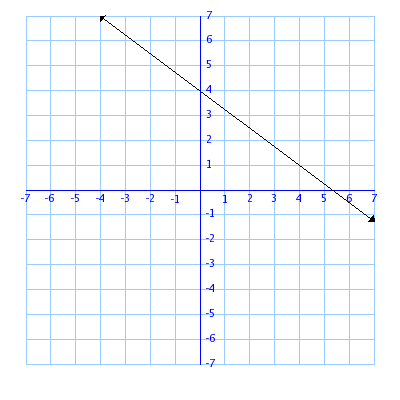-3/4

### 1003/1=3

### 100

A line passes through the points (0,-2) and (3,4) what is the slope?

6/3 = 2/1 = 2

### 200

What is the Y-intercept?

Where the line crosses the Y-axis

### 200

a horizontal line has this kind of slope

zero

### 200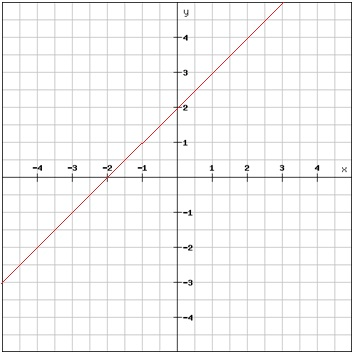1

1

### 200-2/3

### 200

A line passes through the points (2,4) and (5,7) what is the slope?

3/3 = 1

### 300

What letter represents slope?

m

### 300

a line that increases as you move left to right has this kind of slope.

Positive Slope

### 3003/4

### 3004/1 = 4

### 300

A line passes through the points (7,-2) and (-5,-2) what is the slope?

Zero

### 400

What letter represents Y-intercept?

b

### 400

A vertical line has this kind of slope.

Undefined

### 400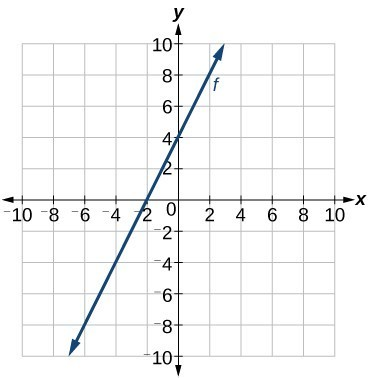4/2 = 2

### 400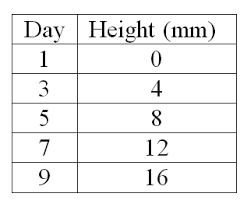4/2 = 2/1 = 2

### 400

A line passes through the points (-2,-1) and (4,3) what is the slope?

4/6 = 2/3

### 500

Write the equation for slope intercept form.

y=mx+b

### 500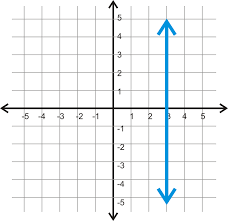Undefined

### 500ZERO!

### 5007/5

### 500

A line passes through the points (8,5) and (8,-7) what is the slope?

Undefined